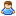1.You are currently browsing our forum as a guest. Create your own forum account to access all forum functionality.

# Unable to take the inverse cosine of a normalized angle

Discussion in 'Programming Questions and Suggestions' started by Aura, Jul 27, 2015.

This last post in this thread was made more than 31 days old.
1. Good morning boys,
I'm trying to troubleshoot a code block and I narrowed it down to this specific function called AimAtTarget (3 of the functions you will see here are ripped off of Aysius' Cruise missile, so please, kudos and credit to him)

The issue I am having currently is that the targetYawAngle variable is causing a stack overflow (I think thats what its called) and is returning a giant fuckoff number (the specific number is 2147483648, the maximum for a double) and I've deduced the reason being that I am somehow taking the inverse cosine of a number greater than one.

However, targetPitchAngle is outputting a perfectly normal and legitimate number, and works flawlessly. The only difference is I am multiplying the normalized vector by 99% of its total value, enough to bring it under and no longer equal to 1.

I even implemented a function called SafeAcos which exists to act as a clamp on the value I am allowing to be Acos'd, however, even after running yawVector through this function the number returned is the same stack overflow.

If you gents could, please take a look at the code below here, and if you know a solution (Or better workaround than adding more 9's to the multiplication vector)

Code:
```void AimAtTarget()
{
//---------- Activate Gyroscopes To Turn Towards Target ----------
Vector3D yawVector = new Vector3D(vTargetPos.GetDim(0), 0, vTargetPos.GetDim(2));
Vector3D pitchVector = new Vector3D(0, vTargetPos.GetDim(1), vTargetPos.GetDim(2));
yawVector.Normalize();
pitchVector = pitchVector.Normalize() * new Vector3D(0.99,0.99,0.99);
//Ok so its the math acos function thats fucking shit up and returning a ginormous number
targetYawAngle = SafeAcos(yawVector.Dot(Z_VECTOR)) * GetMultiplierSign(vTargetPos.GetDim(0));
targetPitchAngle = SafeAcos(pitchVector.Dot(Z_VECTOR)) * GetMultiplierSign(vTargetPos.GetDim(1));

//---------- Set Gyroscope Parameters ----------
SetGyroYaw(targetYawAngle);
SetGyroPitch(targetPitchAngle);
}

double SafeAcos( double x )
{if (x > 1){x = 1;}else if(x < -1){x = -1;}
return Math.Acos(x);}

void SetGyroYaw(double yawRate)
{
for (int i = 0; i < gyroscopes.Count; i++)
{
gyroscopes[i].SetValue(gyroYawField[i], (float)(yawRate * GYRO_FACTOR * gyroYawReverse[i]));
}
}
void SetGyroPitch(double pitchRate)
{
for (int i = 0; i < gyroscopes.Count; i++)
{
gyroscopes[i].SetValue(gyroPitchField[i], (float)(pitchRate * GYRO_FACTOR * gyroPitchReverse[i]));
}
} ```
As an aside, I realize the multipication vector thing doesn't make any sense. I threw it on there while sleep deprived and now I don't exactly know what it's returning because afaik C# doesn't support * being a cross product or dot product so I don't exactly know what it's doing.

In that light, how do i use the Multiply() command? When I go Multiply(yawVector,0.9) I get a compilation error.

2. ### JoeTheDestroyerJunior Engineer

Messages:
573
The * operator between two vectors multiplies values element-wise.

You should note, though, that the Normalize() method is in-place (it changes the object you call it on) and returns a double equal to the length of the original vector. So what you have there is equivalent to:
Code:
```pitchVector = pitchVector.Length() * new Vector3D(0.99,0.99,0.99);
```
Which is probably quite a bit different from what you were expecting. (The Vector3D.Normalize() function does return a vector.) Probably what you wanted was just:
Code:
```pitchVector *= 0.99;
```
As for your main problem, Acos is always troublesome. You've noticed its pickiness w/ out of range numbers, but it also is less accurate than the alternatives. It is usually possible to rework your formulas to use Atan2 instead. If you can, it is much more robust.

3. EDIT: OK now its beginning to actually point towards things, it points at something, misses, and turns back. Guess I need a PID or is there a easier way to control it?

TargetPosition in this case is just vector3D(1,1,1)
Code:
```void AimAtTarget()
{
//---------- Activate Gyroscopes To Turn Towards Target ----------
Vector3D forward = Vector3D.Subtract(vectorPointFront.GetPosition() , vectorPointMid.GetPosition());
forward.Normalize();
Vector3D normal = Vector3D.Subtract(vectorPointTop.GetPosition() , vectorPointMid.GetPosition());
normal.Normalize();
targetVector = Vector3D.Subtract(targetPosition , vectorPointMid.GetPosition());

targetVector = Vector3D.Transform(targetVector, MatrixD.CreateLookAt(POINT_ZERO, forward, normal));
Vector3D pitchVector = new Vector3D( 0, targetVector.GetDim(1), targetVector.GetDim(2) );
Vector3D yawVector = new Vector3D( targetVector.GetDim(0), 0, targetVector.GetDim(2) );

pitchVector.Normalize();
yawVector.Normalize();
targetPitchAngle = Math.Atan2( pitchVector.GetDim(2),pitchVector.GetDim(1) * -1 ) * GetMultiplierSign(targetVector.GetDim(1));
targetYawAngle = Math.Atan2( yawVector.GetDim(0), yawVector.GetDim(2) ) * GetMultiplierSign(targetVector.GetDim(2));

//---------- Set Gyroscope Parameters ----------
SetGyroYaw(targetYawAngle);
SetGyroPitch(targetPitchAngle);
}

double SafeAcos( double x )
{if (x > 1){x = 1;}else if(x < -1){x = -1;}
return Math.Acos(x);}

void SetGyroYaw(double yawRate)
{
for (int i = 0; i < gyroscopes.Count; i++)
{
gyroscopes[i].SetValue(gyroYawField[i], (float)(yawRate * GYRO_FACTOR * gyroYawReverse[i]));
}
}
void SetGyroPitch(double pitchRate)
{
for (int i = 0; i < gyroscopes.Count; i++)
{
gyroscopes[i].SetValue(gyroPitchField[i], (float)(pitchRate * GYRO_FACTOR * gyroPitchReverse[i]));
}
}
int GetMultiplierSign(double value)
{
return (value < 0 ? 1 : -1);
}
```
Editededed

Last edited: Jul 28, 2015
4. I got it facing stuff, implementing a basic PD controller. Thanks bros